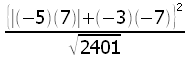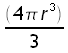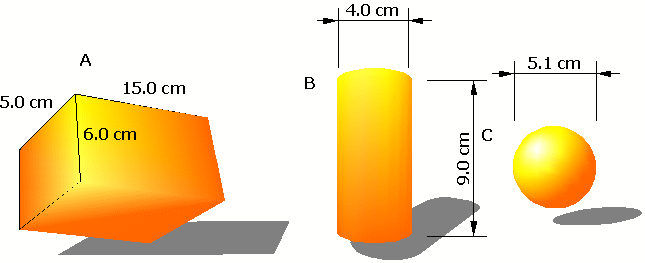MS 095 PreAlgebra 11 February 2005 Quiz 3 Name: _________________________________

1. __________ Make the following calculation:2. __________ Find the volume of the box displayed on the left below. The length is 15 cm, the height is 6 cm, and the width is 5 cm.

3.__________ Find the volume of the cylinder displayed below. The diameter is 4 cm, the length is 9 cm. Use the π (pi) button on your calculator for pi. One point for the correct answer, two points if the answer is rounded to the correct digit.

4. __________ Find the volume of the cylinder displayed below. The diameter is 4 cm, the length is 9 cm. Use the π (pi) button on your calculator for pi. The formula is:

π r² L

One point for the correct answer, two points if the answer is rounded to the correct digit.

5. __________ Find the volume of the sphere displayed below. The diameter is 5.1 cm. Use the π (pi) button on your calculator for pi. The formula is:One point for the correct answer, two points if the answer is rounded to the correct digit.